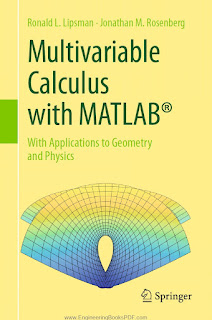CALCULUS MATHEMATICS

# Multivariable Calculus with MATLAB® With Applications to Geometry and Physics Free DownloadThis comprehensive treatment of multivariable calculus focuses on the numerous tools that MATLAB® brings to the subject, as it presents introductions to geometry, mathematical physics, and kinematics. Covering simple calculations with MATLAB®, relevant plots, integration, and optimization, the numerous problem sets encourage practice with newly learned skills that cultivate the reader’s understanding of the material. Significant examples illustrate each topic, and fundamental physical applications such as Kepler’s Law, electromagnetism, fluid flow, and energy estimation are brought to prominent position. Perfect for use as a supplement to any standard multivariable calculus text, a “mathematical methods in physics or engineering” class, for independent study, or even as the class text in an “honors” multivariable calculus course, this textbook will appeal to mathematics, engineering, and physical science students.